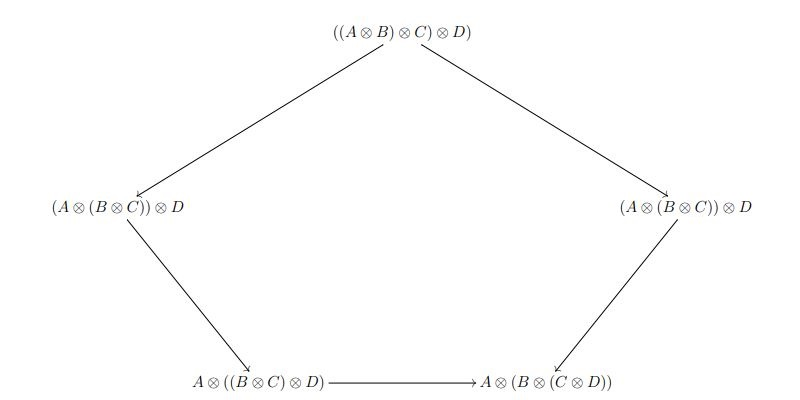# Monoidal bicategories, linear logic and operadsWhen we begin to study mathematics, we learn that the operation of multiplication on numbers satisfies some basic rules. One of these rules, known as associativity, says that for any three numbers a, b and c, we get the same result if we multiply a and b and then multiply the result by c or if we multiply a by the result of multiplying b and c. This leads to the abstract algebraic notion of a monoid, which is a set (in this case the set of natural numbers) equipped with a binary operation (in this case multiplication) that is associative and has a unit (in this case the number 1).

If we continue to study mathematics, we encounter a new kind of multiplication, no longer on numbers but on sets, which is known as Cartesian product. Given two sets A and B, their Cartesian product is the set A x B whose elements are the ordered pairs (a, b), where a is an element of A and b is an element of B. Pictorially, the Cartesian product of two sets is a grid with coordinates given by the elements of the two sets.

This operation satisfies some rules, analogous to those for the multiplication of numbers, but a little more subtle. For example, if we are given three sets A, B and C, then the set A x (B x C) is isomorphic (rather than equal) to the set (A x B) x C. Here, being isomorphic means that we they are essentially the same by means of a one-to-one correspondence between the elements A x (B x C) and those of (A x B) x C.

This construction leads to the notion of a monoidal category, which amounts to a collection of objects and maps between them (in this case the collection of all sets and functions between them) equipped with a multiplication (in this case the Cartesian product) that is associative and has a unit (in this case the one-element set) up to isomorphism.

Monoidal categories, introduced in the '60s, have been extremely important in several areas of mathematics (including logic, algebra, and topology) and theoretical computer science. In logic and theoretical computer science, they connect to linear logic, in which one keeps track of the resources necessary to prove a statement.

This project is about the next step in this sequence of abstract notions of multiplication, which is given by the notion of a monoidal bicategory. In a bicategory, we have not only objects and maps but also 2-maps, which can be thought of as "maps between maps" and allow us to capture how different maps relate to each other. In a monoidal bicategory, we have a way of multiplying their objects, maps and 2-maps, subject to complex axioms.

Monoidal bicategories, introduced in the '90s, have potential for applications even greater than that of monoidal categories, as they allow us to keep track of even more information. We seek to realise this potential by advancing the theory of monoidal bicategories. We will prove fundamental theorems about them, develop new connections to linear logic and theoretical computer science and investigate examples that are of interest in algebra and topology.

Our work connects to algebra via an important research programme known as "categorification", which is concerned with replacing set-based structures (like monoids) with category-based structures (like monoidal categories) in order to obtain more subtle invariants. Our work links to topology via the notion of an operad, which is a flexible tool used to describe algebraic structures in which axioms do not hold as equalities, but rather up to weak forms of isomorphism.

Overall, this project will bring the theory of monoidal bicategories to a new level and promote interdisciplinary research within mathematics and with theoretical computer science.